# Maths - Types

### Type Theory and Set Theory

Type theory and set theory have some similarities as they can be thought of as some sort of container containing a collection of elements but they have important differences:

#### Set Theory

There are various formulations of set theory. In, say the ZF formulation, everything is a set. The elements of a set are themselves sets and their elements are sets. Its sets all the way down until we get to an empty set.

Also an element cannot occur more than once in a set so a given set can't contain more than one empty set.

An element can occur in more than one set but loops would allow paradoxes.

In order to prove things about sets we need a logic layer underpinning it.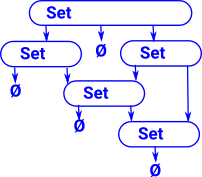#### Type Theory

Elements of a type (terms) are unique to that type, they can't appear outside it. So, for example, the same element can't be a element of two different types.

Elements of a type can optionally be types themselves but, as with sets, a type must not contain itself. That is, it must be a strict hierarchy (no loops). See type universes. Every element has one type, types also have a type, its types all the way up. Again we can't have loops so the types must be in a hierarchy to avoid a paradox like a type of all types.

Proofs can be done within type theory using Curry-Howard correspondence. See pages about equalities. This means we don't need a separate logic to underpin type theory.We can look at types from a mathematical or a computing point of view:

### Types in Computing

In computing we think of type as representing some type of data structure, this determines:

• How the memory is allocated to store the type.
• Type Safety - Allows checks to be made on what operations can be done on the data to provide some level of program validation.
 See these pages for types as related to programming:

With the simpler type theories: types are usually checked at compile time and elements are calculated at runtime. However when we move on to more complicated type theories such as dependent type theory (DTT) this separation get a bit blurred as explained later.

When used in a certain way, types have the same structure as mathematical proofs (see Curry-Howard correspondence). This means that, when coding these structures, in computers, we can use the same machinery to compile the algebra and the proofs. Unlike in set theory which is built over logic.

• Propositions can be represented by types which can only be constructed if they are true. That is an inhabited type can represent a true proposition, an uninhabited type is unproven.
• Proofs can be represented by functions between these types. These functions are not intended to be run (evaluated), the compiler can ensure that it is only possible to compile such a proof if it produces an inhabited type. This gives a proof system that is available at compile-time rather than run-time.

### Types in Mathematics

 Types were originally added to set theory to try to resolve some paradoxes that arose.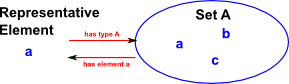Another way to look at it:

A type is the range of significance of a variable, that is, the concept of a variable is tied up with the concept of a type.

## Type Theories

Here are some atomic mathematical types with some examples of terms that may inhabit those types:

Type Term
Boolean True
False

Natural Numbers 0,1,2...
Real Numbers 0.582
Set a

Type is a collection of terms having some common property.

Terms are values (or variables, or functions like the sum of other terms).

We can think of types as classifying values. If we know the type of something we have less information than knowing its value (and also its type). If we know a value without knowing its type, for example 0 (zero) we don't know if it is a natural number, or an integer, or a real number. Hence we don't know what operations might be valid to apply to it.

Types and terms are a similar (but different) concept to sets and elements. In both cases we think about it as a container relationship.

The ':' colon symbol is used to declare the type of a given term:

Type Term
M x:M typing declaration

If the type is not declared, it can be inferred in some circumstances.

We can have a multiple level hierarchy of types. For instance, the type 'M' in the above table may itself have a type of 'Type' ( M:Type ). However giving all higher order types the type of 'Type' could theoretically lead to circular references and hence paradoxes. Some theories allow these 'Type's to be numbered to enforce a hierarchy of universes and prevent any circular references. (see kinds)

## Martin-Löf Type Theories

There are a number of type theories developed by Church, Martin-Löf, etc. Such as:

• STT - Simple Type Theory.
• PTT - Polymorphic Type Theory.
• DTT - Dependent Type Theory.

When working with mathematical types, especially when we are using static typing, we need dependent types. For example vectors and groups can be represented by types that depend on a value. Also fibre bundles are an important concept in mathematics that correspond to dependent types.

So here we will concentrate on dependent type theories and specifically the Martin-Löf and homotopy type theories shown below. These 3 flavours of type theory have different uses, depending on the way properties are preserved under different definitions of equalities.

ETT

Extensional Type Theory

ITT

Intentional Type Theory

HoTT

Homotopy Type Theory

Equality Used Exact equality (after normalisation) Leibniz equality Equivalence
see page here see page here see page here

In M-L extensional type theory two types are the same only if they are defined in exactly the same way. This becomes very important for dependent types, for example, vectors can only be added if they have the same length, however (Vect n) and (Vect (n+0)) would be treated as different types in extensional type theory. This means that extensional type theory limits the calculations and checks that can be done.

In M-L intentional type theory two types are the same if they are defined in the same way or if there is a proof that they are equal. However, in intentional type theory, proofs are not canonical for instance to construct a proof we may have to make expressions more complicated before we can simplify them. So there is not a general algorithm for constructing proofs and each proof required may have to be supplied by a human coder.

In homotopy type theory proofs are canonical (canonicity) and therefore automatic but to get this benefit other things get more complicated (conservation of complexity?). In homotopy type theory structures are built constructively rather than having constraints in the form of equations instead we have rules. The intuition for this type theory comes from homotopy.

### W-Types

For more about W-types see page here.

These types can be defined by the type signatures of constructors only, this allows types to be constructed and proofs to be checked by computers at compile time rather than runtime.

So this is a 'free' construction.

Not all types can be defined in this way but where they are they are initial.

Examples of W-types are Nat, List, Tree.

 For example Nat is defined like this: That is a list of constructors defined recursively. ```data Nat : Type where Z : Nat S : Nat -> Nat```

## Category Theory and Type Theory

We can relate type theory to category theory by linking:

• types to objects
• terms to arrows
 So if a category has various objects each of these could be related to a type.### Constructors and Eliminators

The constructors and eliminators of a type, along with the other constructs here, uniquely specify the type.

For a fuller discussion of Constructors and Deconstructors (Introduction and elimination rules) see the page here.

 There may be many ways that a given type might be constructed:The constructor or constructors for a given type should be the minimum constructor(s) that allows any other possible constructor to be channeled through it.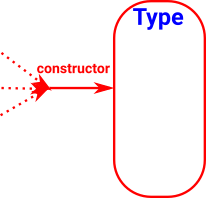The same principles apply to eliminators with the arrows reversed.Constructors and eliminators can also used to prove things about the type. To do this for free objects we require:

• Local soundness of a logical connective - ("Not too strong"/"No junk"). We should not be able to prove more than our basic premises using the introduction and elimination rules of a connective (Logical connectives. The main ones are “and”, “or”, “not” and “implies”).
• Local completeness - ("Not too weak"/"No noise"). We should be able to recover the original premises through the elimination rules of the connective after using the introduction rules.

#### Another way to look at this, in category theory terms

Local soundness and local completeness are the triangle identities of the adjunctions that define logical connectives .

No junk and no noise are also represented by the triangle identities of the free-forgetful adjunction.

### Examples

0 (empty)

A set with zero elements.1 (unit)

A set with one elements.2 (bool)

A set with two elements. This is the data type of Boolean algebra). It is like sum (either) where the terms are not necessarily types.

Like sum (either) the deconstructor requires a type 'C' and map a map from each of the elements to C. In practice we can implement this as a case-statement or even an if-then-else.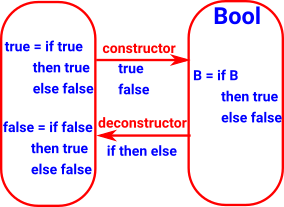Nat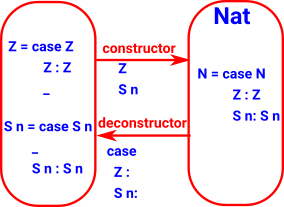List

(free monoid)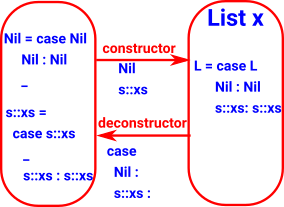Sum +Product ×Function

Constructor (β-conversion) is lambda function, destructor (η-conversion) is function application (substitution).

Related to building and evaluating an expression, lambda calculus adds the concept of binding a variable.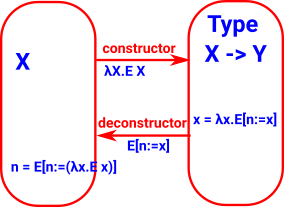where:

• E x is an expression in x
• E[n:=x] is substitute n for x in E
Dependent Sum ΣDependent Product ΠFor every pair of terms there is an identity type. If these two terms are equal the type can be constructed by Rafl

Equality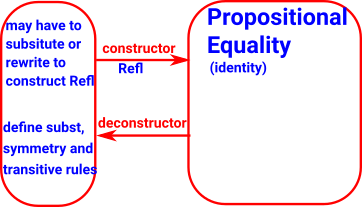Type Name Type Constructor Constructor Eliminator

for more details click on link below

formation constructors deconstructors notes
0
 0:type

 e:0 A:type absurd(e) : A

empty
cannot be constructed
initial object

1
 1:type
 *:1

unit
terminal object

Bool   True
False
conditional expression
if then else

inductive datatypes     induction principle
Nat   Z
S n

elim_Nat: (P : Nat -> Set) -> P Z ->
((k : Nat) -> P k -> P (S k)) ->
(n : Nat) -> P n

where:

• P n = property of number n
• Z = zero
• S n = successor to n

elim_Nat P pz ps Z = pz
elim_Nat P pz ps (S k) = ps k (elim_Nat P pz ps k)

List   cons car
cdr

Pair Pair (a,b) fst
snd

Function   λ function application
Expression Atom
Equality (≡) : t->t->Type refl : { A : Typei } -> ( x : A ) -> x ≡ x

J : { A :Typei } ->
(P: (x y :A) -> x ≡ y -> Typej ) ->
(x . P x x ( refl x )) ->{ x y } . ( eq : x ≡ y ) ->P x y eq

+       sum
Record   tuple projection
×       product - special case
of dependant product
Π       dependant product
space of sections
Σ       dependant sum

For eliminator, for inductive types we can use a recursor.

### Syntax

History: start with type theories with special types for natural numbers and truth values.

(see Lambek&Scott p129)

If A and B are valid types then we can define:

One Element Type Natural Numbers Container for elements of type A Product Truth Value
type notation 1 N PA A×B Ω
term (for type with intuitionistic predicate calculus) * 0,Sn {aA | φ(a)} <a,b> T,, ...
term (for type based on equality) * 0,Sn {aA | φ(a)} <a,b>

aα

a = a'

where:

• φ(a) is a term of type Ω (proof of type A ??, extension of power set ??)

#### Product and Sum Types

Product and sum types construct a type from 2 (or more) types (A and B).

 Product Type Has one constructor (formation) and multiple ways of deconstructing (elimination) (projections).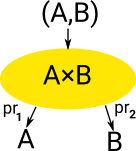Sum Type Has multiple constructors but only one way to use it.In type theory types are defined in terms of: Type formation Term introduction Term Elimination This picture shows how programming language constructs (In this case: Idris) relate to this theory:### Sum, Product and Exponent

A type theory with Sum, Product and Exponent type is related to a Cartesian Closed Category (CCC).

A term may be represented by some variable such as x,y... or a compound term using the following:

If 'M' and 'N' are valid terms, then the following are also valid terms:

Type Term
M[x] We will use this notation to denote a term 'M' with multiple values depending on the value 'x'.
Sum A \/ B <M,N>

This is a union of the two types. An instance will contain an instance of only one of the types.

Product A /\ B (M N)

This contains both of the types (like tuples) . An instance will contain an instance of all of the types.

We can recover the contained types as follows:

• M = π1(M N)
• N = π2(M N)
Exponent A->B λ x:M.N

This is a function from M to N.

where x is an instance of 'M' and the function returns an instance of 'N'

See lambda calculus.

Where:

• M,N are terms, that is a base type or a compound type made up as some combination of the above. That is, they are inductively defined.
• x is an instance of 'M'

Here are some more atomic types, this time denoted in a more category theory way:

Type Term
0 &bottom; empty type (initial)
1 &top; unit type (terminal)
2 True
False
boolean

### Semantics

To put this in a slightly more formal setting see semantics page.

## Type Theory and Logic

There are various approaches to this:

• Curry-Howard correspondence.
• A logic is a logic over a type theory.

## Curry-Howard Correspondence

There is a relationship between computer programs and mathematical proofs, this is known as the Curry-Howard correspondence.

A type in λ-calculus has the same mathematical structure as a proposition in intuitionistic logic.

A constructor for that type corresponds to a proof of the proposition. The following table has some specific types with their corresponding propositions:

Proposition Type
true formula unit type
false formula empty type
implication function type
conjunction product type
disjunction sum type
universal quantification generalised product type (Π type)
existential quantification generalised sum type (Σ type)

#### Logic over a Type Theory

We treat logic as a meta-mathematics, that is a layer above the mathematics that allows us to apply axioms and rules to prove things about the mathematics.

### Type Theory and Information

Informally we sometimes think about 'information' when discussing types. for instance, does some particular operation loose or gain information. The concept of information was precisely defined by Claude Shannon in 1948. It is linked to the concept of 'entropy' which quantifies the amount of uncertainty involved. However something involving probabilities doesn't seem to be what's required here.

 So if we are trying to get an intuitive understanding of why the types/sets on the right are not isomorphic we need to see that the function shown can't be reversed. Intuitively we can see that this function 'looses information'. How can we make this more precise?More specifically, in type theory we have the 'computation rule' (beta reduction rule) and the 'Local completeness rule' (eta reduction rule) - see page here. We say that the 'beta reduction rule' ensures that information is not gained and the 'eta reduction rule' ensures that information is not lost.

One way to think about this is as a constructor as a producer of information (particle of information) and as an evaluator as a consumer (anti-information) which collide and annihilate in computation and local completeness rules.

## Comprehension

Any collection of elements of the same type may form an object of the next higher type.z'x[xz <-> Φ(x)]

## Concepts related to Type## β Reduction

In type theory, β-reduction is a process of 'computation', which generally replaces more complicated terms with simpler ones.

Example of Cut elimination / substitution

In its most general form, β-reduction consists of rules which specify, for any given type T, if we apply an “eliminator” for T to the result of a “constructor” for T, how to “evaluate” the result. (n-Lab)

 (λx.M) N M[N/x]
Function evaluation is called β-reduction. This is done by substitution.

Frank Pfenning - Proof Theory Foundations, Lecture 2

 Here we show deconstruct followed by construct for Product, Sum and Exponent types:## η Reduction

In type theory, η-conversion is a process of 'computation' which is 'dual' to β-conversion.

Whereas β-reduction tells us how to simplify a term that involves an eliminator applied to a constructor, η-reduction tells us how to simplify a term that involves a constructor applied to an eliminator.

In contrast to β-reduction, whose “directionality” is universally agreed upon, the directionality of η-conversion is not always the same. Sometimes one may talk about η-reduction, which (usually) simplifies a constructor–eliminator pair by removing it, but we may also talk about η-expansion, which (usually) makes a term more complicated by introducing a constructor–eliminator pair.

η-reduction is ill-defined for the unit type (n-Lab) .### Examples from Idris

#### Product Type

 Here we start with a product type 'M'. We can deconstruct it by using 'fst' and 'snd' then construct it to get back to 'M' ```Idris> :let M = MkPair 2 "2" Idris> MkPair (fst M) (snd M) (2, "2") : (Integer, String) ``` Here we start with two terms 'x' and 'y' if we first construct using MkPair then deconstruct we get back to 'x' and 'y'. ```Idris> :let x = 3 Idris> :let y = "3" Idris> fst (MkPair x y) 3 : Integer Idris> snd (MkPair x y) "3" : String```

#### Sum Type

 We can't constuct terms as easily because its more difficult to infer the type. ```Idris> :let M = Either Integer String Idris> M Either Integer String : Type Idris> the M (Left 4) Left 4 : Either Integer String Idris> :let m = the M (Left 4) Idris> m Left 4 : Either Integer String ``` 'case' as deconstructor ```lookup_default : Nat -> List a -> a -> a lookup_default i xs def = case list_lookup i xs of Nothing => def Just x => x```

#### Exponent Type

 An element of A->B can be represented as λ a.Φ `λ a.Φ : A->B` β-reduction The second form allows us to change the name of the variable by substitution. Replaces a bound variable in a function body with a function argument - inlining. ```(λ a.Φ) a = Φ (λ a.Φ) x = [a/x]Φ``` η-reduction `f = (λ x . f(x))`

We cam implement in Idris like this:

 We create anominous lambda like this and apply it like this. ```Idris> \x => prim__toStrInt x \x => prim__toStrInt x : Int -> String Idris> (\x => prim__toStrInt x) 3 "3" : String```

### Computations in Idris

A function tends to deconstruct types, rearange and do computations on the terms and then construct the output types.

 The deconstruction tends to be done by a 'case' construct in the form of pattern matching on the left hand side.• Specifically about how constructors and deconstructors relate to introduction and elimination see page here

Where I can, I have put links to Amazon for books that are relevant to the subject, click on the appropriate country flag to get more details of the book or to buy it from them.Computation and Reasoning - This book is about type theory. Although it is very technical it is aimed at computer scientists, so it has more discussion than a book aimed at pure mathematicians. It is especially useful for the coverage of dependant types.